## Derivative Surface White Paper

We are introducing a new and extrodinarily powerful paradigme for surface construction. One of the major building blocks for this paradigm is the "Derivative Surface". The derivative surface captures information about the tangent (first derivative), radius of curvature (second derivative), change in radius of curvature (third derivative), and optionally even higher order derivatives. Traditionally this information is just derived from a curve on surface and is more or less fixed. In our paradigm the derivative surface can not only be constructed without a base surface but it can be edited using scaling, rotation, direction reversal, etc. Instead of specifying a lot of curves to construct and constrain a surface, the new paradigm allows complex surface construction and manipulation with just a few curves and their corresponding derivative surface definition.

The derivative surface is primarily used in blending and sweeping where the tangency, curvature and change in curvature is important to match between surfaces. To make high quality surfaces, "Class A Surfaces", it is important to match up not only tangency but higher order derivatives.

The derivative surface can be defined in many different ways. This white paper will explore how the derivative surface is constructed. Look at the Blending White Paper to see how the derivative surface is used to create surfaces.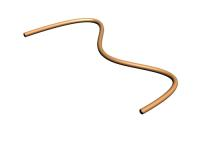Parallel to Vector

A derivative surface is a surface which runs along a curve. Often a tangency along the curve can be simply specified as "Parallel to a Vector". The two images to the right show two derivative surfaces parallel to the vector {0,0,1} with 30 unit and 120 unit first derivative length. The length of the derivative controls how strong the corresponding tangency is for any subsequent surface constructions.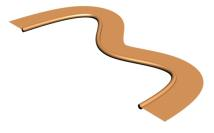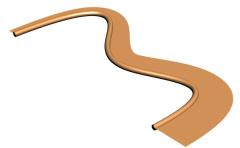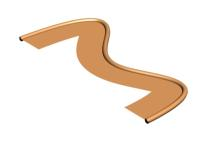Purpendicular to Vector with Scaling and Orientation Flip

Surface tangency can also be specified as "Purpendicular to a Vector". In the case to the left the derivative surface is purpendicular to the vector {0,0,1}. The strength of the tancency can be scaled uniformly along the curve as shown in the middle image above. The tangency can also be flipped as shown in the right image above.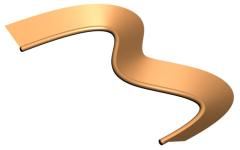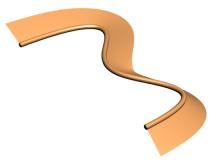Purpendicular to Vector with Single Angle and Two Angle Rotation

The angle of the tangency can be controled by a start and end rotation angle. The rotation angle rotates the tangent field at each point along the curve by a value interpolated from a start and end angle which are specified. In the case to the left above, the angle is 30 degrees for both. In the case to the right above, the start angle is 30 degrees and the end angle is -45 degrees.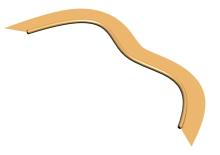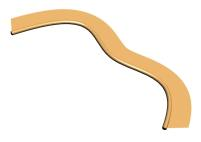Flair Angle Start and End

The "Flair Angle" of the ends of the derivative surface can also be controled. The flair angle is the rotation of the curve in the plane defined by curve tangent and the dervative surface.Radius of Curvature with Constant and Variable Radius

The radius of curvature can also be specified directly. In the case to the left a radius of 60 is specified. The case to the right starts at 60 and goes to 15. You can clearly see how the radius effects the shape of the derivative surface.Flip Radius of Curvature

The radius of curvature can be flipped to either direction relative to the tangency.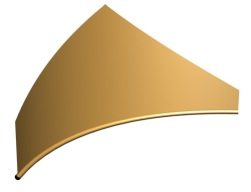Three Morphs Applied to Derivative Surface

The user has the ability to apply morphs at any point along the curve to change the strength, orientation, and curvature of the tangent field. The first image above shows 3 morphs applied at 0%, 50% and 100% along the curve with a linear blend between the morphs. The second image shows a degree 2 blend between the morphs.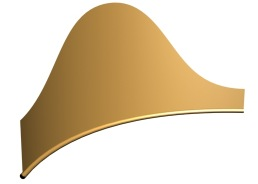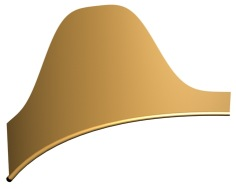These two images show degree 3 and degree 4 blending between the 3 morph values shown above.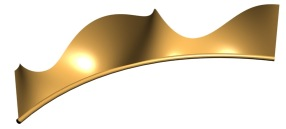This image shows five morph values applied to a curve with different tangent strengths and rotations. As you can see the derivative field morphing allows very explicit and fine control over the derivative field.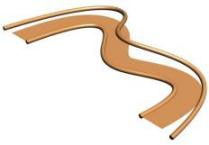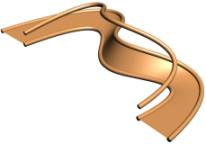Before and After using a G1 Curve With and Without Specified Tangent Length

For more precise control over the derivative surface tangency it is possible to specify a G1 curve. You can see that the G1 curve controls the direction of the tangent field of the derivative surface. If a tangent length is not specified the G1 curve will also control the length of the tangency as seen in the right most image.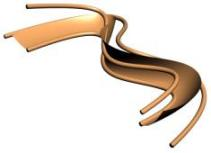The Radius of Curvature Controled By G2 Curve

The Radius of Curvature can also be precisely controled using a curve. The radius of curvature is defined as the distance between the original curve and the G2 Curve. You can see two examples above where the G2 curve has been modified to show the effect on the Derivative Surface.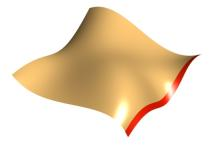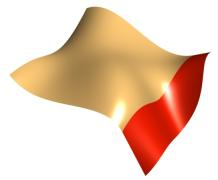Derived From Surface Tangent

The derivative surface can also be derived from a base surface. The same controls in terms of the length of the tangent and scaling can be used as shown above. The above images show a 30 and 120 length derivative in the left two images. The right image shows a scaling of 2 applied to the 120 length case along the curve.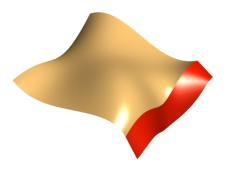Rotation of the Tangent Field using Constant and Variable Rotation

The angle of the tangent field relative to the original surface can be controled by specifying a start and end angle of rotation. In the case to the left you can see a 30 degree rotation along the entire curve. In the case to the right the angle starts at 0 degrees and goes to 50 degrees along the curve.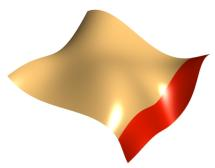Higher Order Derivatives Derived From Surfaces

Higher order derivatives of a surface can be specified. The left image shows the standard tangent field which is G1. The second image shows G2 where the radius of curvature matches. The right image shows G3 where the radius of curvature and the change in radius of curvature match.

This is just a simple overview of the construction techniques used for Derivative Surfaces. The main thing is that it gives you a flexable and powerful set of tools to specify derivative information along a curve that can be subsequently used to construct surfaces that maintain that derivative information.